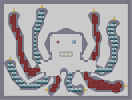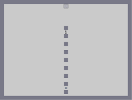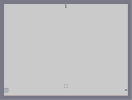### Octopus Pulling The Finger 8 Times

This map has been removed from listings by the author.Hover over the thumbnail for a full-size version.

Author paradox-dragon author:paradox-dragon drone mine n-art octopus rated tileset 2011-06-09 2013-01-19 5 by 5 people. \$Octopus Pulling The Finger 8 Times#paradox-dragon#n-art#00000000000000;LH00000000;QQLH0000000P00LH000000P0000LH00000:O000400000:MQJ000QQQQLH0FJ00400000000FJOOOO000LH0200P0000000000000FJ000LH20P000000000000000FJ00E?0P00000GKQQQQQQQJ8700AC0P00;QQ000MQQQJ00FJ0E?00A00P00OMI00000FJ000AC0E000:MI0000GKLH00P00Q00A000000000;QQQQ<;0000MI;Q<0000000P0000000000LK005000000?P000000000000O50000000CP00000000;IFI0000000001P00000000:OKQLH0000000BP00000000000O0N0000000>P000000000MI0B0@0000000P00000000N000C0D0000000:OOOO=:000LH0:O=00000000FJMI00P0000LH0000;LH0000000GK000M@00@000P00QQQLHGK00000@D00D000:OOMJ00GOOOMIB0DB00N00000000FQI00000>0N>00N000000GOH000000GK0E0P0N00000;000FJLOOK000A0B0N00000P0OH0000000MI00C00@0000:50FLOOMQQI0000P00A0000000000000000000:O=000000000000000000000000|12^348,252!12^348,246!12^342,252!12^354,252!0^240,48!0^246,48!0^258,48!0^264,48!0^252,42!0^252,36!0^252,30!0^96,72!0^90,72!0^84,72!0^78,72!0^72,72!0^84,66!0^84,60!0^84,54!0^552,72!0^570,72!0^576,72!0^564,54!0^288,540!0^48,360!0^54,360!8^444,252,1!6^444,252,5,0,0,1!7^372,300,1!7^396,300,1!7^420,300,1!7^444,324,2!7^420,348,3!7^396,348,3!7^372,348,3!7^348,324,0!12^348,258!12^240,420!12^228,432!12^228,420!12^228,408!12^216,408!12^216,420!12^216,432!12^216,444!12^204,456!12^204,444!12^204,432!12^204,420!12^204,408!12^192,420!12^192,432!12^192,444!12^192,456!12^180,456!12^180,444!12^180,432!12^180,420!12^180,408!12^168,408!12^168,420!12^168,432!12^168,444!12^168,456!12^156,432!12^144,420!12^156,420!12^156,408!12^144,408!12^144,396!12^156,396!12^168,396!12^168,384!12^168,372!12^156,372!12^156,384!12^144,384!12^132,384!12^120,372!12^132,372!12^144,372!12^156,360!12^144,360!12^132,360!12^120,360!12^120,348!12^132,348!12^144,348!12^144,336!12^132,336!12^120,336!12^108,336!12^108,324!12^120,324!12^132,324!12^144,324!12^132,312!12^120,312!12^108,312!12^108,300!12^120,300!12^132,300!12^132,288!12^120,288!12^108,288!12^108,276!12^120,276!12^132,276!12^132,264!12^120,264!12^108,264!12^108,252!12^120,252!12^132,252!12^132,240!12^120,240!12^108,240!12^132,228!12^120,228!12^108,228!12^96,228!12^96,216!12^108,216!12^120,216!12^132,216!12^120,204!12^108,204!12^96,204!12^96,192!12^108,192!12^120,192!12^120,180!12^108,180!12^108,168!12^96,168!12^96,180!12^84,180!12^84,192!12^72,180!12^72,168!12^84,168!12^96,156!12^84,156!12^72,156!12^60,144!12^72,144!12^84,144!12^96,144!12^96,132!12^84,132!12^72,132!12^60,132!12^60,120!12^84,120!12^72,120!12^96,120!12^96,108!12^84,108!12^72,108!12^60,108!12^60,96!12^72,96!12^84,96!12^96,96!12^96,84!12^84,84!12^72,84!12^348,456!12^360,468!12^348,468!12^336,468!12^336,480!12^348,480!12^360,480!12^360,492!12^348,492!12^336,492!12^336,504!12^348,504!12^348,516!12^336,516!12^336,528!12^324,504!12^324,516!12^312,516!12^312,528!12^324,528!12^336,540!12^324,552!12^324,540!12^312,552!12^312,540!12^300,528!12^300,540!12^300,552!12^516,432!12^504,444!12^516,444!12^528,444!12^504,456!12^516,456!12^528,456!12^504,468!12^516,468!12^528,468!12^540,468!12^552,468!12^516,480!12^528,480!12^540,480!12^552,480!12^564,480!12^576,480!12^588,480!12^600,480!12^612,480!12^624,492!12^636,492!12^648,492!12^648,504!12^660,504!12^672,504!12^684,504!12^696,504!12^696,492!12^708,492!12^720,492!12^720,504!12^732,504!12^732,516!12^732,528!12^720,528!12^720,540!12^708,552!12^696,552!12^684,552!12^720,516!12^708,504!12^708,516!12^708,528!12^708,540!12^696,540!12^696,528!12^696,516!12^684,516!12^684,528!12^684,540!12^672,540!12^672,528!12^672,516!12^660,516!12^660,528!12^660,540!12^648,540!12^648,528!12^648,516!12^636,504!12^636,516!12^636,528!12^636,540!12^624,540!12^624,528!12^624,516!12^624,504!12^612,492!12^612,504!12^612,516!12^612,528!12^612,540!12^600,540!12^600,528!12^600,516!12^600,504!12^600,492!12^588,492!12^588,516!12^588,504!12^588,528!12^588,540!12^576,540!12^576,528!12^576,516!12^576,504!12^576,492!12^564,492!12^564,504!12^564,516!12^564,528!12^552,528!12^552,516!12^552,504!12^552,492!12^540,492!12^528,492!12^528,504!12^540,504!12^540,516!12^540,528!12^528,516!12^564,360!12^576,348!12^564,348!12^552,348!12^552,336!12^564,336!12^576,336!12^588,336!12^588,324!12^576,324!12^564,324!12^552,324!12^564,312!12^576,312!12^588,312!12^588,300!12^576,300!12^576,288!12^588,288!12^588,276!12^576,276!12^588,264!12^576,252!12^588,252!12^600,240!12^588,240!12^576,240!12^576,228!12^588,228!12^600,228!12^600,216!12^588,216!12^576,216!12^564,216!12^576,204!12^564,204!12^588,204!12^600,204!12^588,192!12^576,192!12^564,192!12^564,180!12^576,180!12^576,168!12^564,168!12^564,156!12^576,156!12^576,144!12^564,144!12^588,132!12^588,144!12^588,120!12^588,108!12^588,96!12^576,96!12^576,84!12^564,84!12^552,84!12^552,96!12^564,96!12^552,108!12^564,108!12^576,108!12^576,120!12^576,132!12^564,132!12^564,120!12^552,120!12^552,132!12^90,84!12^78,84!12^72,90!12^78,90!12^84,90!12^90,90!12^96,90!12^90,96!12^78,96!12^66,96!12^60,102!12^60,114!12^60,126!12^60,138!12^72,138!12^72,126!12^72,114!12^72,102!12^66,102!12^66,108!12^66,114!12^66,120!12^66,126!12^66,132!12^66,138!12^66,144!12^78,144!12^90,144!12^90,138!12^84,138!12^78,138!12^78,132!12^78,126!12^78,120!12^78,114!12^78,108!12^78,102!12^84,102!12^90,108!12^96,102!12^90,102!12^90,114!12^84,114!12^96,114!12^90,120!12^90,126!12^84,126!12^96,126!12^90,132!12^96,138!12^96,150!12^90,150!12^84,150!12^78,150!12^72,150!12^78,156!12^90,156!12^96,162!12^90,162!12^84,162!12^78,162!12^72,162!12^78,168!12^90,168!12^102,168!12^114,168!12^120,174!12^120,186!12^120,198!12^120,210!12^126,216!12^132,222!12^132,234!12^132,246!12^132,258!12^132,270!12^132,282!12^132,294!12^132,306!12^138,312!12^72,174!12^78,192!12^78,186!12^78,180!12^78,174!12^84,174!12^90,174!12^90,180!12^90,186!12^84,186!12^90,192!12^96,186!12^96,174!12^102,174!12^108,174!12^114,174!12^114,180!12^102,180!12^102,186!12^108,186!12^114,186!12^114,192!12^102,192!12^102,198!12^108,198!12^114,198!12^96,198!12^102,204!12^114,204!12^114,210!12^108,210!12^102,210!12^96,210!12^102,216!12^114,216!12^126,222!12^120,222!12^114,222!12^108,222!12^102,222!12^96,222!12^102,240!12^102,234!12^102,228!12^108,234!12^114,228!12^126,228!12^126,234!12^120,234!12^114,234!12^114,240!12^126,240!12^126,246!12^120,246!12^114,246!12^108,246!12^114,252!12^126,252!12^126,258!12^120,258!12^114,258!12^108,258!12^108,270!12^114,264!12^126,264!12^126,270!12^120,270!12^114,270!12^126,276!12^114,276!12^108,282!12^114,282!12^120,282!12^126,282!12^126,288!12^114,288!12^108,294!12^114,294!12^120,294!12^126,294!12^126,300!12^114,300!12^108,306!12^114,306!12^120,306!12^126,306!12^138,318!12^138,318!12^132,318!12^120,318!12^126,312!12^126,318!12^126,318!12^120,312!12^114,318!12^114,306!12^114,312!12^120,324!12^126,330!12^132,336!12^144,348!12^144,360!12^144,378!12^150,390!12^156,390!12^156,396!12^156,378!12^150,372!12^150,372!12^144,366!12^138,360!12^138,354!12^138,348!12^138,348!12^138,342!12^138,330!12^138,330!12^132,330!12^120,330!12^120,324!12^120,324!12^114,324!12^114,324!12^120,336!12^126,336!12^132,342!12^144,384!12^144,384!12^156,402!12^162,414!12^168,426!12^174,420!12^180,414!12^186,414!12^192,414!12^198,414!12^198,420!12^198,426!12^198,432!12^192,438!12^186,444!12^186,444!12^198,444!12^204,438!12^204,438!12^210,444!12^216,444!12^216,438!12^210,426!12^210,426!12^198,432!12^186,426!12^180,426!12^174,432!12^174,426!12^174,420!12^150,408!12^150,408!12^156,420!12^162,420!12^156,414!12^150,420!12^150,420!12^150,432!12^150,432!12^162,432!12^162,426!12^156,426!12^150,420!12^162,402!12^162,402!12^162,396!12^162,396!12^162,390!12^162,390!12^162,378!12^162,372!12^162,372!12^162,366!12^126,342!12^126,360!12^126,366!12^126,366!12^126,360!12^126,354!12^126,366!12^126,378!12^132,378!12^138,378!12^138,372!12^132,372!12^126,372!12^108,318!12^126,324!12^138,324!12^144,330!12^138,336!12^114,336!12^114,330!12^108,330!12^144,342!12^144,354!12^120,342!12^126,348!12^120,354!12^138,354!12^132,354!12^132,360!12^138,366!12^132,366!12^132,366!12^120,366!12^126,384!12^138,384!12^150,360!12^162,360!12^156,366!12^150,366!12^168,378!12^162,384!12^150,378!12^150,384!12^144,390!12^150,396!12^150,402!12^144,402!12^168,390!12^168,402!12^162,408!12^150,414!12^144,414!12^150,426!12^174,414!12^168,414!12^174,408!12^186,420!12^186,408!12^204,414!12^210,408!12^222,408!12^234,408!12^234,414!12^240,414!12^240,426!12^234,432!12^234,420!12^234,426!12^228,426!12^222,426!12^222,432!12^222,432!12^222,420!12^228,414!12^222,414!12^216,414!12^210,414!12^210,420!12^216,426!12^204,426!12^192,426!12^210,432!12^210,438!12^198,438!12^186,432!12^216,450!12^210,450!12^198,456!12^198,450!12^210,450!12^204,450!12^192,450!12^186,450!12^186,456!12^186,438!12^180,438!12^174,438!12^168,438!12^168,450!12^180,450!12^180,444!12^174,444!12^174,456!12^174,450!12^342,456!12^354,456!12^354,462!12^348,462!12^342,462!12^336,462!12^342,468!12^354,468!12^360,474!12^300,546!12^300,534!12^306,528!12^306,534!12^306,540!12^306,546!12^306,552!12^318,552!12^318,546!12^318,534!12^318,528!12^318,522!12^318,510!12^318,516!12^330,510!12^330,528!12^330,534!12^330,534!12^336,540!12^360,486!12^354,504!12^354,498!12^354,492!12^354,486!12^354,480!12^354,474!12^348,474!12^342,474!12^336,474!12^342,480!12^342,486!12^336,486!12^348,486!12^342,492!12^342,504!12^342,498!12^348,498!12^336,498!12^348,510!12^348,522!12^348,528!12^342,528!12^342,522!12^342,516!12^342,510!12^336,510!12^336,522!12^330,522!12^324,522!12^330,516!12^330,504!12^324,510!12^318,504!12^312,510!12^312,522!12^330,552!12^336,546!12^336,534!12^330,540!12^330,546!12^324,546!12^324,534!12^318,540!12^312,528!12^312,546!12^312,534!12^558,360!12^570,360!12^570,348!12^570,336!12^570,324!12^570,312!12^558,312!12^552,330!12^552,342!12^552,354!12^582,336!12^582,324!12^582,318!12^582,312!12^582,306!12^582,300!12^582,294!12^582,288!12^582,282!12^582,276!12^582,270!12^582,258!12^588,258!12^582,264!12^588,270!12^588,282!12^588,294!12^588,306!12^588,318!12^576,306!12^576,294!12^576,282!12^582,252!12^588,330!12^582,330!12^576,330!12^570,330!12^564,330!12^558,336!12^558,330!12^558,324!12^558,318!12^564,318!12^570,318!12^576,318!12^576,354!12^570,354!12^564,354!12^558,354!12^558,348!12^558,342!12^570,342!12^564,342!12^576,342!12^594,246!12^588,246!12^582,246!12^576,246!12^594,252!12^594,258!12^594,240!12^582,240!12^576,234!12^582,234!12^588,234!12^594,234!12^600,234!12^600,222!12^594,222!12^594,228!12^576,228!12^582,228!12^582,222!12^576,222!12^588,222!12^600,210!12^594,192!12^594,198!12^594,204!12^594,210!12^594,216!12^588,210!12^582,210!12^582,216!12^570,216!12^570,210!12^564,210!12^576,210!12^582,204!12^582,198!12^582,192!12^588,198!12^576,204!12^570,198!12^576,198!12^564,198!12^570,204!12^570,192!12^570,186!12^564,186!12^576,192!12^570,180!12^570,168!12^570,156!12^570,144!12^570,132!12^570,120!12^570,114!12^570,108!12^570,108!12^570,102!12^570,102!12^576,186!12^576,174!12^570,174!12^564,174!12^564,156!12^564,156!12^564,162!12^570,156!12^570,162!12^576,162!12^576,162!12^576,138!12^570,144!12^570,144!12^570,144!12^576,150!12^570,150!12^570,150!12^564,144!12^564,138!12^564,132!12^570,132!12^576,132!12^576,138!12^588,132!12^588,132!12^576,132!12^582,138!12^582,138!12^582,120!12^582,120!12^582,114!12^582,114!12^582,120!12^582,126!12^582,126!12^582,108!12^582,108!12^576,102!12^576,96!12^564,84!12^570,90!12^570,90!12^564,90!12^558,90!12^564,96!12^564,108!12^564,96!12^564,96!12^564,108!12^558,108!12^552,102!12^558,102!12^558,114!12^558,126!12^564,126!12^564,132!12^564,138!12^558,138!12^558,144!12^558,132!12^552,126!12^552,114!12^558,120!12^564,114!12^564,102!12^558,96!12^552,90!12^558,84!12^570,84!12^576,90!12^582,96!12^570,96!12^582,102!12^588,102!12^588,114!12^576,114!12^588,126!12^588,132!12^588,132!12^588,138!12^570,138!12^570,126!12^576,126!12^582,132!12^564,150!12^582,144!12^510,432!12^522,432!12^522,438!12^522,444!12^522,456!12^522,462!12^522,474!12^522,474!12^522,480!12^522,468!12^522,450!12^528,438!12^528,450!12^528,462!12^528,474!12^516,438!12^510,438!12^504,438!12^516,450!12^510,450!12^510,444!12^504,450!12^510,456!12^510,462!12^516,462!12^516,474!12^510,474!12^510,480!12^510,468!12^504,462!12^534,462!12^534,456!12^540,462!12^546,462!12^546,468!12^558,474!12^558,468!12^564,474!12^570,474!12^570,480!12^588,480!12^582,480!12^594,480!12^606,480!12^534,468!12^534,474!12^540,474!12^546,474!12^552,474!12^558,480!12^546,480!12^534,480!12^528,486!12^528,498!12^528,510!12^546,528!12^558,528!12^570,528!12^576,534!12^582,540!12^582,534!12^582,528!12^582,528!12^582,528!12^582,522!12^582,516!12^582,516!12^582,510!12^582,510!12^582,504!12^582,498!12^582,492!12^582,486!12^582,486!12^582,486!12^570,486!12^570,486!12^564,486!12^558,486!12^552,486!12^546,486!12^540,486!12^540,486!12^534,486!12^534,486!12^534,492!12^534,498!12^534,498!12^534,504!12^534,510!12^540,516!12^540,522!12^540,522!12^540,516!12^540,516!12^540,510!12^546,504!12^546,498!12^546,498!12^546,498!12^558,498!12^558,498!12^564,498!12^570,498!12^570,504!12^570,510!12^570,522!12^570,522!12^558,516!12^552,516!12^558,504!12^558,504!12^552,510!12^546,510!12^546,510!12^546,516!12^546,516!12^552,522!12^558,516!12^558,516!12^564,522!12^564,522!12^552,522!12^552,528!12^546,522!12^564,504!12^558,522!12^534,522!12^534,516!12^540,498!12^546,492!12^552,498!12^558,492!12^570,492!12^576,486!12^576,498!12^558,510!12^564,510!12^576,510!12^570,516!12^576,522!12^732,510!12^732,522!12^726,528!12^726,522!12^726,516!12^726,504!12^726,510!12^720,510!12^720,522!12^720,534!12^720,498!12^714,498!12^708,498!12^702,498!12^696,498!12^702,492!12^714,492!12^714,504!12^702,504!12^720,546!12^702,552!12^690,552!12^672,546!12^684,546!12^678,546!12^690,546!12^696,546!12^702,546!12^708,546!12^714,546!12^714,540!12^702,540!12^690,540!12^678,540!12^672,534!12^678,540!12^678,534!12^684,534!12^690,534!12^696,534!12^702,534!12^708,534!12^714,534!12^714,528!12^702,528!12^690,528!12^678,534!12^678,528!12^672,522!12^672,510!12^678,504!12^690,504!12^678,510!12^678,522!12^678,516!12^684,522!12^684,510!12^690,510!12^690,516!12^690,522!12^696,522!12^696,510!12^714,510!12^714,516!12^714,522!12^702,522!12^708,522!12^702,516!12^702,510!12^708,510!12^666,540!12^666,534!12^666,528!12^666,522!12^666,516!12^666,510!12^666,504!12^654,504!12^654,510!12^660,510!12^660,522!12^654,522!12^654,516!12^654,528!12^654,534!12^654,540!12^660,534!12^642,540!12^642,534!12^648,534!12^648,522!12^648,510!12^648,498!12^642,492!12^630,492!12^624,486!12^618,486!12^612,486!12^606,486!12^600,486!12^594,486!12^588,486!12^594,492!12^606,492!12^624,492!12^618,492!12^642,498!12^642,504!12^642,510!12^642,510!12^636,516!12^636,516!12^636,516!12^636,516!12^642,522!12^642,522!12^636,516!12^642,516!12^642,516!12^636,498!12^630,498!12^624,498!12^624,498!12^612,498!12^606,498!12^606,498!12^594,498!12^588,498!12^618,498!12^600,498!12^618,504!12^630,504!12^636,510!12^642,528!12^636,522!12^636,528!12^636,534!12^636,534!12^606,504!12^594,504!12^588,510!12^588,522!12^588,522!12^588,528!12^588,534!12^630,540!12^618,540!12^606,540!12^594,540!12^594,534!12^606,534!12^600,534!12^594,528!12^594,522!12^594,516!12^594,510!12^600,510!12^600,522!12^612,534!12^612,522!12^612,510!12^624,510!12^624,522!12^624,534!12^624,534!12^636,528!12^636,528!12^630,516!12^630,516!12^630,528!12^630,528!12^630,528!12^630,510!12^630,522!12^630,534!12^606,522!12^606,516!12^606,516!12^606,504!12^606,516!12^606,528!12^606,510!12^618,510!12^618,522!12^618,522!12^618,528!12^618,528!12^618,534!12^618,516!12^147,424!12^147,429!12^209,454!12^214,453!12^198,411!12^191,412!12^122,378!12^123,382!12^166,366!12^165,363!12^141,320!12^138,315!12^141,315!12^118,171!12^75,184!12^77,190!12^76,187!12^99,232!12^100,237!12^596,198!12^597,195!12^554,137!12^556,141!12^580,257!12^580,263!12^580,268!12^580,272!12^579,257!12^553,319!12^554,314!12^506,473!12^507,478!12^530,521!12^535,526!12^532,524!12^619,482!12^622,482!12^717,549!12^711,548!12^676,548!12^682,548!0^408,318!0^408,324!0^408,330!0^384,318!0^384,324!0^384,330!1^372,324!1^396,324!1^420,324!6^252,60,5,0,0,1!6^252,84,5,0,0,1!6^228,84,5,0,0,1!6^228,108,5,0,0,1!6^252,108,5,0,0,1!6^252,132,5,0,0,1!6^228,132,5,0,0,1!6^228,156,5,0,0,1!6^228,180,5,0,0,1!6^204,204,5,0,0,1!6^204,180,5,0,0,1!6^204,228,5,0,0,1!6^204,252,5,0,0,1!6^204,276,5,0,0,1!6^204,300,5,0,0,1!6^204,324,5,0,0,1!6^228,324,5,0,0,1!6^228,348,5,0,0,1!6^204,348,5,0,0,1!6^228,372,5,0,0,1!6^276,468,5,0,0,1!6^276,492,5,0,0,1!6^252,492,5,0,0,1!6^228,492,5,0,0,1!6^228,516,5,0,0,1!6^204,516,5,0,0,1!6^180,516,5,0,0,1!6^156,516,5,0,0,1!6^132,516,5,0,0,1!6^132,492,5,0,0,1!6^108,492,5,0,0,1!6^108,468,5,0,0,1!6^84,468,5,0,0,1!6^84,444,5,0,0,1!6^84,420,5,0,0,1!6^60,420,5,0,0,1!6^60,396,5,0,0,1!6^84,396,5,0,0,1!6^60,372,5,0,0,1!6^420,444,5,0,0,1!6^420,468,5,0,0,1!6^420,492,5,0,0,1!6^396,492,5,0,0,1!6^420,516,5,0,0,1!6^420,540,5,0,0,1!6^444,540,5,0,0,1!6^468,540,5,0,0,1!6^492,540,5,0,0,1!6^588,420,5,0,0,1!6^612,420,5,0,0,1!6^612,444,5,0,0,1!6^636,420,5,0,0,1!6^660,420,5,0,0,1!6^660,396,5,0,0,1!6^660,372,5,0,0,1!6^684,372,5,0,0,1!6^684,348,5,0,0,1!6^684,324,5,0,0,1!6^684,300,5,0,0,1!6^684,276,5,0,0,1!6^708,276,5,0,0,1!6^708,252,5,0,0,1!6^684,252,5,0,0,1!6^660,252,5,0,0,1!6^660,228,5,0,0,1!6^684,228,5,0,0,1!6^708,228,5,0,0,1!6^684,204,5,0,0,1!6^660,204,5,0,0,1!6^660,180,5,0,0,1!6^684,180,5,0,0,1!6^660,156,5,0,0,1!6^684,156,5,0,0,1!6^684,132,5,0,0,1!4^240,66,1!4^252,66,1!4^264,66,1!4^264,90,1!4^252,90,1!4^228,90,1!4^228,114,1!4^240,114,1!4^252,114,1!4^258,114,1!4^252,138,1!4^240,138,1!4^228,138,1!4^216,138,1!4^216,162,1!4^228,162,1!4^240,162,1!4^234,186,1!4^228,186,1!4^216,186,1!4^204,186,1!4^204,210,1!4^216,210,1!4^216,234,1!4^204,234,1!4^204,258,1!4^216,258,1!4^216,282,1!4^204,282,1!4^204,306,1!4^216,306,1!4^204,330,1!4^216,330,1!4^228,330,1!4^240,330,1!4^240,354,1!4^228,354,1!4^216,354,1!4^228,378,1!4^240,378,1!4^239,90,1!4^48,378,1!4^60,378,1!4^72,378,1!4^84,402,1!4^72,402,1!4^60,402,1!4^48,402,1!4^54,426,1!4^60,426,1!4^72,426,1!4^84,426,1!4^96,426,1!4^96,450,1!4^84,450,1!4^72,450,1!4^78,474,1!4^84,474,1!4^96,474,1!4^108,474,1!4^120,474,1!4^102,498,1!4^108,498,1!4^120,498,1!4^132,498,1!4^144,498,1!4^126,522,1!4^132,522,1!4^144,522,1!4^156,522,1!4^168,522,1!4^180,522,1!4^192,522,1!4^204,522,1!4^216,522,1!4^228,522,1!4^234,522,1!4^228,498,1!4^216,498,1!4^240,498,1!4^252,498,1!4^264,498,1!4^276,498,1!4^282,498,1!4^288,474,1!4^276,474,1!4^264,474,1!4^408,450,1!4^420,450,1!4^432,450,1!4^408,474,1!4^420,474,1!4^426,474,1!4^396,498,1!4^408,498,1!4^420,498,1!4^408,522,1!4^420,522,1!4^432,522,1!4^432,546,1!4^444,546,1!4^456,546,1!4^468,546,1!4^480,546,1!4^492,546,1!4^420,546,1!4^576,426,1!4^588,426,1!4^600,426,1!4^612,426,1!4^624,426,1!4^636,426,1!4^648,426,1!4^660,426,1!4^666,426,1!4^612,450,1!4^648,402,1!4^660,402,1!4^672,402,1!4^648,378,1!4^660,378,1!4^672,378,1!4^684,378,1!4^690,378,1!4^672,354,1!4^684,354,1!4^696,354,1!4^696,330,1!4^684,330,1!4^672,330,1!4^672,306,1!4^684,306,1!4^696,306,1!4^684,282,1!4^672,282,1!4^696,282,1!4^708,282,1!4^708,258,1!4^696,258,1!4^684,258,1!4^672,258,1!4^660,258,1!4^660,234,1!4^672,234,1!4^684,234,1!4^696,234,1!4^708,234,1!4^690,210,1!4^684,210,1!4^672,210,1!4^660,210,1!4^654,210,1!4^654,234,1!4^648,186,1!4^660,186,1!4^672,186,1!4^684,186,1!4^690,162,1!4^684,162,1!4^672,162,1!4^660,162,1!4^648,162,1!4^672,138,1!4^684,138,1!4^696,138,1!0^84,54!0^84,60!0^84,66!0^84,72!0^90,72!0^96,72!0^72,72!0^78,72!0^252,30!0^240,48!0^252,36!0^252,42!0^246,48!0^252,48!0^264,48!0^564,54!0^564,66!0^564,72!0^558,72!0^552,72!0^564,60!0^672,120!0^678,120!0^684,120!0^690,120!0^696,120!0^684,114!0^684,108!0^684,102!0^504,528!0^504,534!0^504,540!0^510,540!0^516,540!0^522,540!0^504,546!0^504,552!0^744,528!0^744,522!0^744,516!0^750,516!0^756,516!0^762,516!0^744,510!0^744,504!0^288,552!0^288,546!0^288,534!0^288,528!0^276,540!0^282,540!0^270,540!0^60,360!0^66,360!0^72,360!0^60,354!0^60,348!0^60,342!0^60,348!0^60,348# N-art

## Other maps by this authorSoar The Fall

## Comments

Pages: (0)

### pretty nice

better than any n-art ive ever made, i haven't made any n-art tho... still pretty freakin AWSOME

R5TED!!!

### One of

the best n-arts this year, hands down

### Don't use tiles to make the outlines

just a rule of thumb. :)
funny n-art :)
well... since you used tiles to make the outline, you should have made it much more smooth... just saying :P